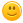def f(n):
if n<=3:
return n-3
else:
return f(n+2)+2*n
n=int(input())
print(f(n))

answered Oct 2 by (40,320 points)
edited Oct 3 by Peter Minarik

I do not know what you're doing here, but something is clear: your recursion will blow up.

Your function f() seems to be used in recursion. This means it must have usually 2 paths: one, to keep calling itself (recursion) and one for termination.

Your termination condition is n to be <= 3.

However, in the recursion part, you keep increasing n for the next iteration by 2.

It's easy to see that your recursion will never terminate (for n > 3 start values) as then n just keeps growing and growing and never reaches the termination condition, i.e. to be <= 3. (Note, it actually reaches the termination condition after n overflows.)

commented Oct 5 by (100 points)

Correct code is this ...

def f(n):
if n<=3:
return n-3
else:
return(n+2)+2*n
n=int(input("Enter No : "))
print(f(n))

May this help you ...commented Oct 6 by (40,320 points)
The original poster didn't explain what s/he was after.

I don't think we can make any assumptions about what the *correct code* is without knowing what the program is supposed to do. :)